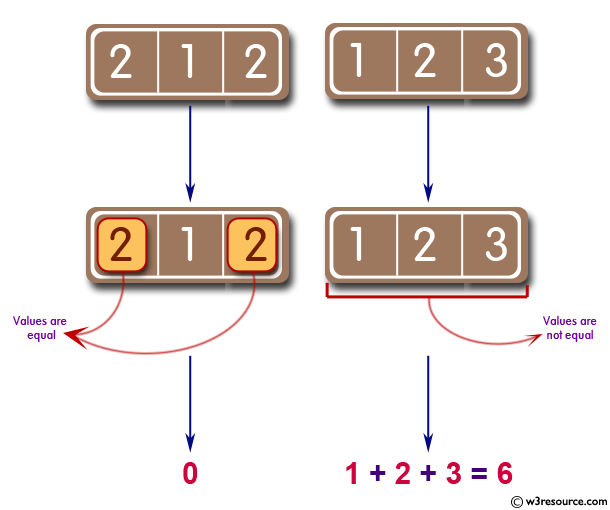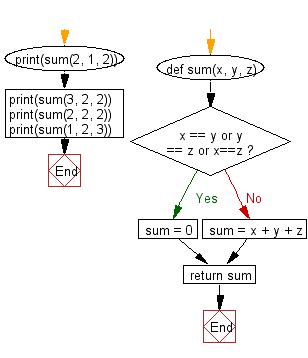﻿ Python: Sum of three given integers. However, if two values are equal sum will be zero - w3resource# Python: Sum of three given integers. However, if two values are equal sum will be zero

## Python Basic: Exercise-33 with Solution

Write a Python program to sum of three given integers. However, if two values are equal sum will be zero.

Pictorial Presentation:Sample Solution:-

Python Code:

``````def sum(x, y, z):
if x == y or y == z or x==z:
sum = 0
else:
sum = x + y + z
return sum

print(sum(2, 1, 2))
print(sum(3, 2, 2))
print(sum(2, 2, 2))
print(sum(1, 2, 3))
```
```

Sample Output:

```0
0
0
6
```

Flowchart:## Visualize Python code execution:

The following tool visualize what the computer is doing step-by-step as it executes the said program:

Python Code Editor:

Have another way to solve this solution? Contribute your code (and comments) through Disqus.

What is the difficulty level of this exercise?

Test your Python skills with w3resource's quiz

﻿

## Python: Tips of the Day

Try-catch-else construct:

```try:
foo()
except Exception:
print("Exception occured")
else:
print("Exception didnt occur")
finally:
print("Always gets here")
```